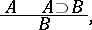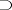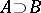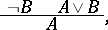# Modus ponens

Jump to: navigation, search

law of detachment, rule of detachment

A derivation rule in formal logical systems. The rule of modus ponens is written as a schemewhereanddenote formulas in a formal logical system, andis the logical connective of implication. Modus ponens allows one to deducefrom the premise(the minor premise) and(the major premise). Ifandare true in some interpretation of the formal system, thenis true. Modus ponens, together with other derivation rules and axioms of a formal system, determines the class of formulas that are derivable from a set of formulasas the least class that contains the formulas fromand the axioms, and closed with respect to the derivation rules.

Modus ponens can be considered as an operation on the derivations of a given formal system, allowing one to form the derivation of a given formulafrom the derivationofand the derivationof.

#### Comments

The more precise Latin name of the law of detachment is modus ponendo ponens. In addition there is modus tollendo ponens, which is written as the schemewherestands for negation anddenotes the logical "or" .